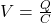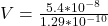Two plates with area 7.00×10−3 m27.00×10−3 m2 are separated by a distance of 4.80×10−4 m4.80×10−4 m . If a charge of 5.40×10−8 C5.40×10−8 C

Question

Two plates with area 7.00×10−3 m27.00×10−3 m2 are separated by a distance of 4.80×10−4 m4.80×10−4 m . If a charge of 5.40×10−8 C5.40×10−8 C is moved from one plate to the other, calculate the potential difference (voltage) between the two plates. Assume that the separation distance is small in comparison to the diameter of the plates.

in progress 0
5 months 2021-08-27T12:25:07+00:00 1 Answers 0 views 0

The voltage is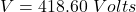Explanation:

From the question we are told that

The area of the both plate is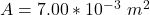The distance between the plate is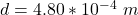The magnitude of the charge is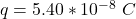The capacitance of the capacitor that consist of the two plates is mathematically represented as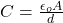Where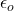is the permitivity of free space with a value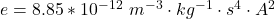So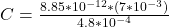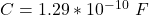The potential difference between the plate is mathematically represented as